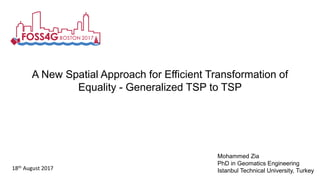Successfully reported this slideshow.

# A New Spatial Approach for Efficient Transformation of Equality - Generalized TSP to TSP

1

Share×
1 of 46
1 of 46

# A New Spatial Approach for Efficient Transformation of Equality - Generalized TSP to TSP

1

Share

The Equality - Generalized Travelling Salesman Problem (E-GTSP) asks to find a Hamiltonian cycle visiting each group exactly once, where each group represents a type of visiting node. This can represent a range of combinatorial optimization problem of NP-hard type like planning, logistics, etc. Its solution requires transformation of E-GTSP to TSP before solving it using a given TSP solver. This paper presents 5 different search-algorithms for optimal transformation which considers spatial spread of nodes of each group. Algorithms are tested over 15 cities with different street-network’s fractal-dimension for 5 instances of group-counts each. It’s observed that the R-Search algorithm, which selects nodes from each group depending upon their radial separation with respect to the start-end point, is the optimal search criterion among all other algorithms with a mean length error of 8.8%. This study will help developers and researchers to answer complex routing problems from a spatial perspective.

The Equality - Generalized Travelling Salesman Problem (E-GTSP) asks to find a Hamiltonian cycle visiting each group exactly once, where each group represents a type of visiting node. This can represent a range of combinatorial optimization problem of NP-hard type like planning, logistics, etc. Its solution requires transformation of E-GTSP to TSP before solving it using a given TSP solver. This paper presents 5 different search-algorithms for optimal transformation which considers spatial spread of nodes of each group. Algorithms are tested over 15 cities with different street-network’s fractal-dimension for 5 instances of group-counts each. It’s observed that the R-Search algorithm, which selects nodes from each group depending upon their radial separation with respect to the start-end point, is the optimal search criterion among all other algorithms with a mean length error of 8.8%. This study will help developers and researchers to answer complex routing problems from a spatial perspective.

## More Related Content

### Related Books

Free with a 30 day trial from Scribd

See all

### Related Audiobooks

Free with a 30 day trial from Scribd

See all